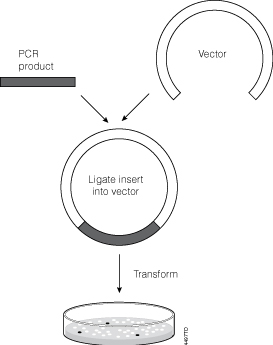# Successful Ligation and Cloning of Your InsertYou have PCR amplified your insert of interest, made sure the PCR product is A tailed and are ready to clone into a T vector (e.g., pGEM®-T Easy Vector). The next step is as simple as mixing a few microliters of your purified product with the cloning vector in the presence of DNA ligase, buffer and ATP, right? In fact, you may need to consider the molar ratio of T vector to insert.

After the insert DNA is prepared for ligation, estimate the concentration by comparing the staining intensity of your PCR product with that of DNA molecular weight standard of similar size and known concentrations on an ethidium bromide-stained agarose gel. If the vector DNA concentration is unknown, estimate the vector concentration by the same method. Test various vector:insert DNA ratios to determine the optimal ratio for a particular vector and insert. In most cases, a 1:1 or 1:3 molar ratio of vector:insert works well, but you may want to consider 1:5, 5:1 and even a 10:1 ratio. The following example illustrates the calculation of the amount of insert required at a specific molar ratio of vector:insert.

[(ng of vector × kb size of insert) ÷ kb size of vector] × (molar amount of insert ÷ molar amount of vector) = ng of insert

Example:
How much 500bp insert DNA needs to be added to 100ng of 3.0kb vector in a ligation reaction for a desired vector:insert ratio of 1:3?
[(100ng vector × 0.5kb insert) ÷ 3.0kb vector] × (3 ÷ 1) = 50ng insert

Our BioMath Calculator is an easy way to calculate the molar ratio of vector to insert for ligation.

The vector:insert ratio changes, depending on the insert, even if you use the same vector. If you use the same vector:insert ratio for many different inserts and the insert size increases or decreases, recalculate the amount of insert needed for ligation using the equation above or our handy BioMath Calculator to ensure the molar ratio stays the same.

For more information on cloning, consult the Cloning chapter of the Protocols and Applications Guide.

The following two tabs change content below.1.Abduljalal says: